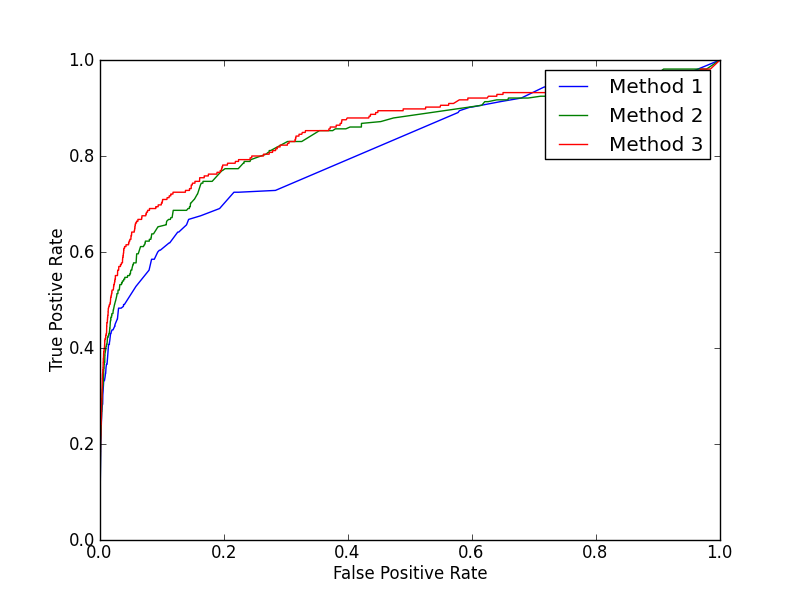# Explaining how ROC curve works# Explaining how ROC curve works

Receiver Operating Characteristic Curve or ROC Curve is a great tool to evaluate the performance of a classification model. Measuring the overall quality of a model cannot be left trivial. A classification model maps the features of the input data into probabilities to fall in different categories, so instead of saying this input looks like a dog or cat, the model outputs a probability value X which could be described as – this input comes under a specific class or category with a confidence score of X% percentage. To keep it more accurate, we set some threshold i.e. T and we say if the probability is greater than or equal to T, the input falls under this category else to the other category. For instance, we built a spam classifier which maps the inputs into two classes like Yes and No /spam or not spam and we set our threshold T to 0.50, so we assume that any probability greater than or equal to 0.50 would fall into spam category, otherwise into the other category. Our model predicted a score 0.756 and it could be described as, the model is certain that, the current input falls into Yes / Spam category with a confidence score of 0.756. For every binary classifier, there are four possible outcomes in total namely True Positives (TP), True Negatives (TN), False Positives (FP) and False Negatives (FN). A True Positive is an outcome where the model correctly predicts the positive class. Similarly, a True Negative is an outcome where the model correctly predicts the negative class. A False Positive is an outcome where the model incorrectly predicts the positive class. And a False Negative is an outcome where the model incorrectly predicts the negative class.

ROC Curve visualizes the distinguishing ability of a classifier at various thresholds. It plots two parameters:

• True Positive Rate
• False Positive Rate

True Positive Rate (TPR) is a synonym for recall and is therefore defined as follows:

Similarly, False Positive Rate (FPR) is defined as:

Lowering the classification threshold would classify more inputs to True Positives, thus increasing both TPR and FPR. A typical ROC Curve would look like the below.

This could be evaluated as a performance measure of three different classifiers at various classification thresholds. It would be really inefficient calculate the ROC values when we deal with millions of data points. One solution for this is called Area Under the Curve or AUC Curve.

## Area Under the Curve or AUC

AUC measures the entire two dimensional area underneath the ROC Curve from (0,0) to (1,1). We could have to shade the whole area under the ROC Curve to see how it would look like. Hold on, I’ve already done that for you.

ROC is a probability curve and AUC represents the degree or measure of separability between classes. We conclude, higher the AUC, better the model. In our example, higher the AUC, better the model in classifying data points between spam and not spam.

## Interpreting AUC

A perfect model would have AUC score of 1.0 which in turn could categorize spam email into spam class and non-spam email into no spam class. A model which has AUC score somewhere around 0.0 would be really worst in action i.e. it fails thoroughly to discern between classes. When AUC turns to be 0.5, it means every data point has 50 – 50 chance to be in either classes, which is shit.